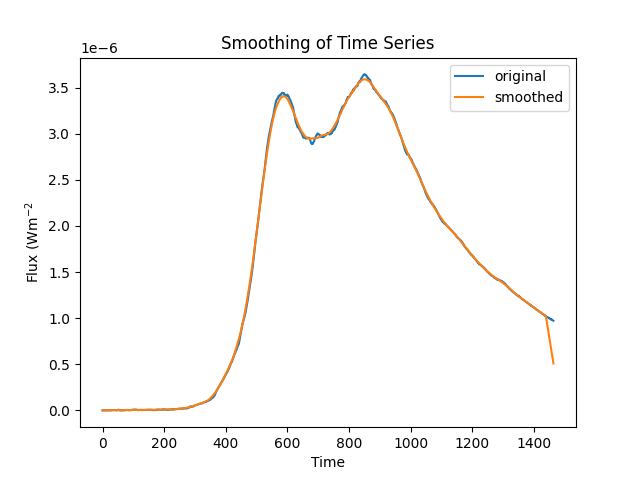# Smoothing of timeSeries data using convolution filters#

How to smooth a TimeSeries using a convolution filter kernel from convolution and convolve function.

import matplotlib.pyplot as plt

from astropy.convolution import Box1DKernel, convolve

from sunpy.data.sample import GOES_XRS_TIMESERIES
from sunpy.timeseries import TimeSeries


Let’s first create a TimeSeries from sample data.

goes_lc = TimeSeries(GOES_XRS_TIMESERIES).truncate('2011/06/07 06:10', '2011/06/07 07:00')


Now we will extract data values from the TimeSeries and apply a BoxCar filter to get smooth data. Boxcar smoothing is equivalent to taking our signal and using it to make a new signal where each element is the average of w adjacent elements. Here we will use astropy’s convolve function with a “boxcar” kernel of width w = 10.

goes_lc = goes_lc.add_column(
'xrsa_smoothed',
convolve(goes_lc.quantity('xrsa'), kernel=Box1DKernel(50))
)


Plotting original and smoothed timeseries.

fig, ax = plt.subplots()
ax.set_xlabel('Time')
ax.set_ylabel("Flux (Wm$^{-2}$")
ax.set_title('Smoothing of Time Series')
ax.plot(goes_lc.quantity('xrsa'), label='original')
ax.plot(goes_lc.quantity('xrsa_smoothed'), label='smoothed')
ax.legend()
plt.show()Total running time of the script: (0 minutes 0.478 seconds)

Gallery generated by Sphinx-Gallery